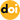### Optimal modulation strategy based on fundamental reactive power for dual‑active‑bridge converters

Vol. 21, No. 12, pp. 1780-1792, Dec. 202110.1007/s43236-021-00319-4

###### Abstract

Dual-active-bridge (DAB) converters are widely used in bidirectional power transmission and voltage conversion. At present, phase-shift (PS) control is one of the most common and mature control methods for DAB converters. To improve the efficiency and performance of DAB converters, the soft switching and current stress are optimized by increasing the inner phaseshift ratio. However, under different PS controls, it is difficult to establish a unified mathematical model using the traditional instantaneous integral method. To solve this problem, based on the approximate equivalence of the fundamental component of a high-frequency link decomposed by Fourier series, an optimal fundamental modulation strategy is proposed, which takes the fundamental reactive power as the objective of optimization. The trajectories for each of the electrical parameters are analyzed intuitively through a vector diagram of the phasor. A practical optimal control strategy scheme for engineering is obtained. Finally, the effectiveness of the theoretical analysis and the proposed method are verified by experimental results.

###### Statistics
Show / Hide Statistics

Cumulative Counts from September 30th, 2019
Multiple requests among the same browser session are counted as one view. If you mouse over a chart, the values of data points will be shown.